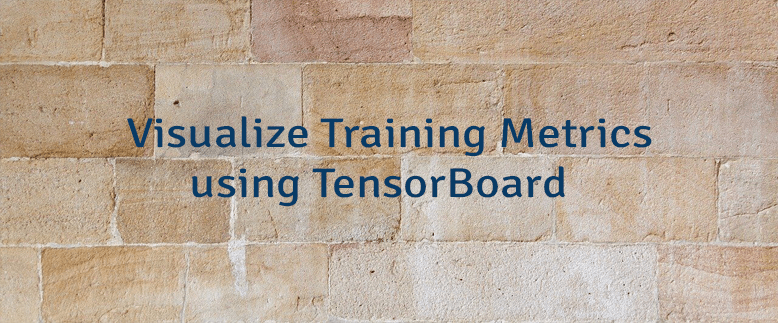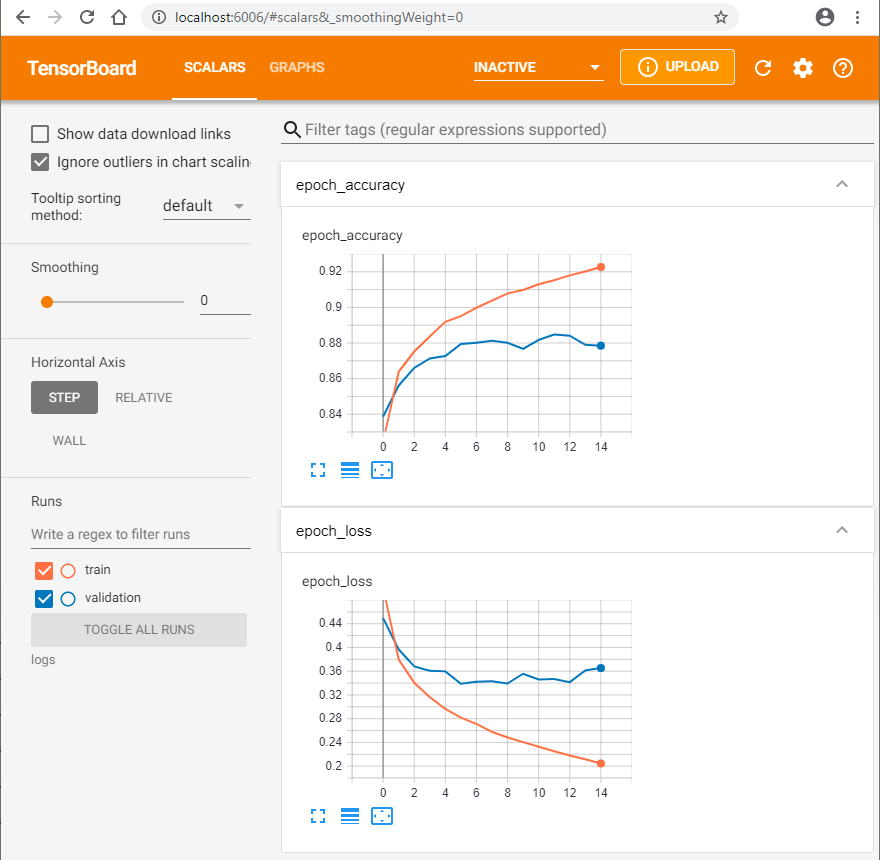# Visualize Training Metrics using TensorBoard

TensorBoard is a tool which allows visualizing training metrics (e.g. loss and accuracy), model graph, activation histograms, profiling results, etc.

This tutorial demonstrates how to visualize training metrics using TensorBoard. TensorFlow 2 depends on TensorBoard. So we only need to install `tensorflow` using `pip` package manager from the command line.

``pip install tensorflow``

We created a model that is used to classify images. It consists of a sequence of one `Flatten` layer and two `Dense` layers. We passed `metrics` argument to the `compile` method in order to report the accuracy of the training. We created an instance of the `TensorBoard` class. A constructor has the `log_dir` parameter which defines the path of the directory where to save the log files. An instance of `TensorBoard` are passed to the `fit` method as `callbacks` argument. We use 15 epochs to train the model.

``````from tensorflow import keras

fashionMnist = keras.datasets.fashion_mnist
(trainImages, trainLabels), (testImages, testLabels) = fashionMnist.load_data()

trainImages = trainImages / 255
testImages = testImages / 255

model = keras.Sequential([
keras.layers.Flatten(input_shape=(28, 28)),
keras.layers.Dense(128, activation='relu'),
keras.layers.Dense(10, activation='softmax')
])

model.compile(
loss='sparse_categorical_crossentropy',
metrics=['accuracy']
)

tensorBoard = keras.callbacks.TensorBoard(log_dir='logs')

model.fit(
trainImages,
trainLabels,
epochs=15,
validation_data=(testImages, testLabels),
callbacks=[tensorBoard]
)``````

After we trained the model, we can start TensorBoard from the command line. We can provide the log directory with `logdir` option.

``tensorboard --logdir logs``

The command will inform that TensorBoard has started. By default, it is served at `http://localhost:6006`.

Once you open a browser, you can see the TensorBoard dashboard. It has tabs in the top navigation bar. If "Scalars" tab is selected, we can see the training metrics. In our case, dashboard displays a loss and accuracy for each epoch. The orange curves show the metrics for the training set and the blue curves shows the metrics for the validation set.